## 动态规划解决背包问题

背包问题(Knapsack problem)是一个动态规划问题，假设有n种货物，每种货物的的价值是v[i],重量是w[i],需要在背包负载有限的前提下求出具有最大货值的组合（策略），使用暴力算法也可以求出背包问题最优解，而利用动态规划可以将算法的复杂度降至接近于多项式复杂度，背包问题根据每种货物的数量限制可分为以下几种：

0-1背包问题：每种货物数量1件，选择每种货物的策略是取(1)还是不取(0)。

1.1、概念介绍

线性规划为静态规划，而动态规划显著的特点是问题有阶段性，以下面的最短路线问题为例：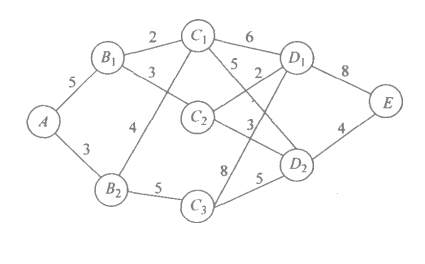以上介绍了动态规划中状态、决策、状态转移方程、策略的概念，接下来再看与策略优化相关的概念，对于最短路线问题而言，最优的策略显然要求各个阶段路程之和最小。假设在k阶段状态为sk，采用了决策uk后得到k+1阶段的状态sk+1,用一个阶段指标函数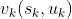来量化决策uk，例如从A到B1节点有：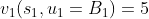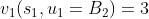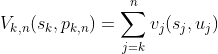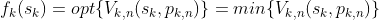1.2、逆推法

求解动态规划常用方法有逆推法和顺推法，设动态规划问题一共有n个阶段，逆推法从n阶段向前逆向推导每个阶段的最优决策，直至推导出最优指标函数f1(s1),然后利用已推导的最优决策，从开始阶段顺次得到各个阶段最优状态。以开头最短路线问题为例：k=3时：

f3(C1)=min{6+f4(D1),5+f4(D2)}=min{6+8,5+4}=9,从C1出发到下个阶段最优决策是选择D2，记u3(C1)=D2，在确保最短短路程的前提下，有状态转移方程D2=T(C1,u3(C1))

f3(C2)=min{2+f4(D1),3+f4(D2)}=min{2+8,3+4}=7,记u3(C2)=D2。

f3(C3)=min{8+f4(D1),5+f4(D2)}=min{8+8,5+4}=9,记u3(C3)=D2

k=2时：

f2(B1)=min{2+f3(C1),3+f3(C2)}=min{2+9,3+7}=10,记u2(B1)=C2

f2(B2)=min{4+f3(C1),5+f3(C3)}=min{4+9,5+9}=13,记u2(B2)=C1

k=1时：

f1(A)=min{5+f2(B1),3+f2(B2)}=min{5+10,3+13}=15,记u1(A)=B1

k=1时，由u1(A)=B1，确定路线A到B1

k=2时，由u2(B1)=C2，确定路线B1C2

k=3时，由u3(C2)=D2，确定路线C2D2

k=4时，由u4(D2)=E，确定路线D2E

1.3、顺推法

顺推法顾名思义是从k=1阶段开始顺序推导至k=n阶段，首先引入一个新的最优指标函数：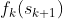C1同时连接B1和B2B1、B2是第一阶段结束状态可选集合，同时B1、B2又是第二阶段开始状态集合；而C1属于第三阶段开始状态也是第二阶段结束状态之一。根据定义有：f2(C1)=min{2+f1(B1),4+f1(B2)},逆推法的最优指标函数以开始状态作为标识，而顺推法的最优指标函数以结束状态作为标识，选择的标识不同是因两者算法差异造成的，用公式可表达为：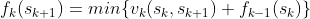①式中sk是一个变量，sk+1是一个定值，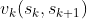表示状态sksk+1之间的阶段指标函数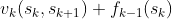表达的是一个集合，公式中含义可根据f2(C1)的表达式对号入座来理解，特别的，设初始状态f0(s1)=0。顺推法求解上面最短路线问题即为求解最优指标函数f4(E)，接下来用顺推法解决之前的最短路线问题。

k=1时，有

f1(B1)=5,u1(B1)=A;f1(B2)=3,u1(B2)=A

k=2时，有

f2(C1)=min{2+f1(B1),4+f1(B2)}=min{2+5,4+3}=7,u2(C1)=B1B2

f2(C2)=min{3+f1(B1)}=8，u2(C2)=B1

f2(C3)=min{5+f1(B1)}=8，u2(C3)=B2

k=3时，有

f3(D1)=min{6+f2(C1),2+f2(C2),8+f2(C3)}=min{6+7,2+8,8+8}=10

u3(D1)=C2

f3(D2)=min{5+f2(C1),3+f2(C2),5+f2(C3)}=min{7+7,3+8,7+8}=10

u3(D2)=C2

k=4时

f4(E)=min{8+f3(D1), 4+f3(D2)}=min{8+10,4+11}=15,u4(E)=D2

u4(E)=D2 ，u3(D2)=C2u2(C2)=B1 ，u1(B1)=A

可根据目标最优指标函数来区分使用的是逆推还是顺推法，如目标函数是形式f1(A)，即以初始状态和初始阶段为最优指标函数标识符的，使用的是逆推法；而类似f4(E)以最终阶段和最终状态为函数标识符的是顺推法。

2.1、完全背包问题

前面介绍过，完全背包问题指的是每种货物数量不限，以下面这个问题为例，假设一辆汽车最多可运7吨货物，有甲、乙、丙三种物资，每种物资货值及重量如下表：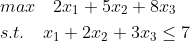x1,x2,x3都是件数、皆为整数。对物资甲、乙、丙分三阶段分配数量，起始状态s1≤7代表待分配吨位不大于7吨，x1,x2,x3件数作为决策变量，分配完物资甲后第二阶段初始状态s2=s1-x1,与此类似，分配完物资乙后第三阶段状态s3=s2-2x2,分配完丙后s4=s3-3x3=0，有了状态转移方程之后引入最优指标函数fk(s),其意义指在装载能力不大于s的前提下，k阶段到最后第3阶段的最大货值，显然f1(7)的值即为所求的最大货值，由目标最优指标函数形式可知，将使用逆推法求解该问题,f1(7)表达式为：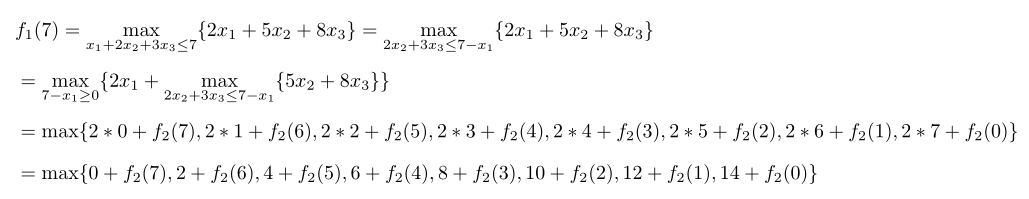f1(7)中根据状态转移方程s2=s1-x1确定了第二阶段可分配重量，接下来逐个分析其中多项式，以f2(7)为例，f2(7)代表在第一阶段货物甲分配了0件：

-免费试读结束-

 下一篇 隐马尔科夫链HMM详解 评论区